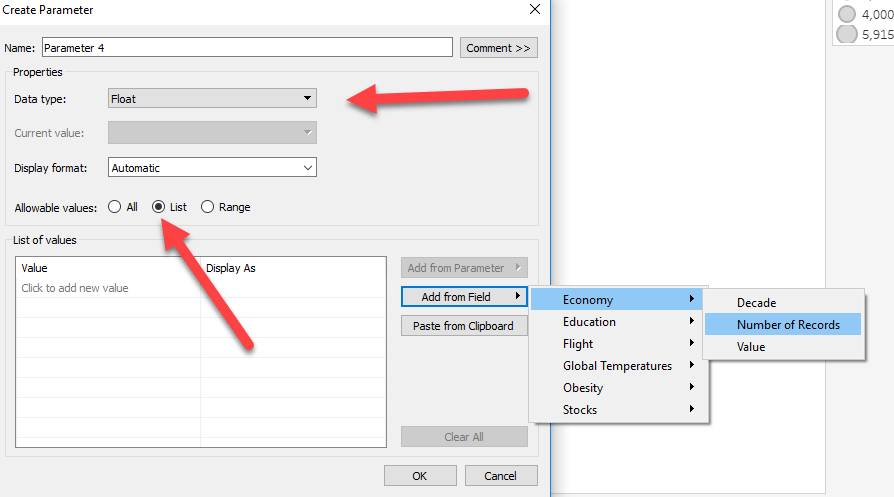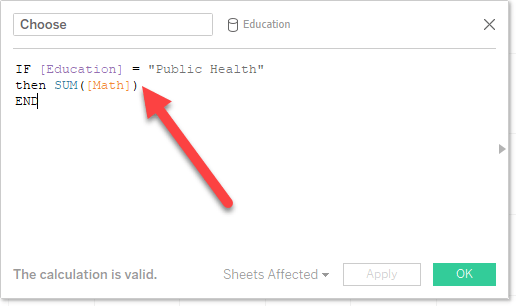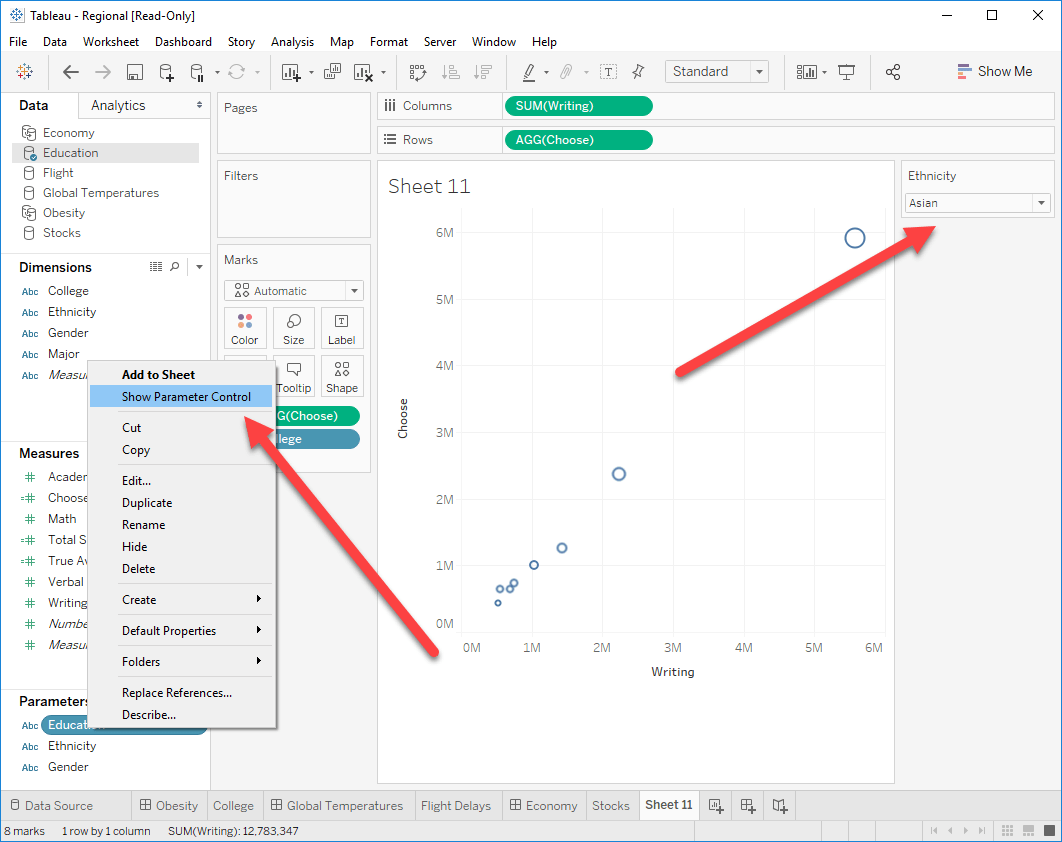# How to Use a Parameter in Tableau

## How to Use a Parameter

Step 1:  Create a Parameter

Step  2:  Attach it to a calculation

Step 3: Use Parameter Controls

Lets get started:

You can create a parameter by right clicking the measures plane.  When you do this , you will have a choice of data types for your parameter. For this example we will use a string.You need to choose the data type of the parameter. Name the parameter.You can enter the values you want and how you want them to be displayed.The last step will be use the parameter in a calculation.Once the new parameter is linked to a calculation. It will work as a dynamic selector.Now that you know how to create a parameter. You see the multiple uses of it below.

## 8 Ways to Use Parameters in Tableau

1. Dynamic X axis or Y axis: In tableau using parameter user can change the measure of X axis or Y axis. This is very helpful in case when user is unsure about which measure user want to see in any axis. Also, it provide the flexibility to user to analyze the same chart with different axis.
• Example: Consider a scatter chart, where X axis is Sales, but Y axis can be Discount, Profit or Quantity.
1. Dynamic reference line: Similarly like dynamic axes, user can create dynamic reference line. To create a dynamic reference line in tableau, user needs to create a integer or floating parameter with fixed lower and upper value and then use this parameter as a reference line. After that user can change the value of the parameter to change the value of reference line.
1. Filtering with parameter: Parameters can also be used to filter the data. Also, it provides user the flexibility to change the filter dimension depending upon the selection value of filter.
• Example: Consider the case where the requirement is to filter the data either on product or sub-category or category. So in this case instead of providing 3 different filter for each type, using parameter and calculation (based on parameter) user can provide on only filter to user.
1. Filtering Top/Bottom N rows: Using normal filters only fixed number of values can be shown like Top 10 or more. But, using parameter user can select Top N rows where N is : 10,20,30… etc.
1. Use of parameter across different data sources: Filters in tableau are specific to data sources but parameters are common to all data sources and can be reused across the workbook.
• Example: Suppose, I have bar chart across countries from data source1 and another scatter chart showing counties across sales and profit from data source2. Now in this case if user would like to apply filter which is common to both charts then using parameter it can be done.
1. Create dynamic bins for histogram: To create a histogram user needs to specify the number of bins for the histogram. This number of bins is a fixed number by default. But, using parameter user can dynamically change the number of bins and histogram will get updated accordingly
1. Table calculations using parameter: In tableau using table calculations user can calculate the moving average of any of the measure. Moving average is majorly used in predictive analysis. Also, while calculating the moving average, user needs to provide number if previous values that is needed to be considered to calculate the moving average. Now, using parameter user can dynamically select the number of previous values that is needed to be considered and based on that the analysis will get change.
2. Hide/Unhide the sheet using parameter: Using parameter in tableau use[wp-svg-icons icon=”code” wrap=”i”]r can hide/unhide the sheet. This is a most widely used case of parameter as multiple time the scenario comes where user needs to hide or unhide a sheets depending upon requirement.
• Example: Suppose there are two sheets named as “A” and “B”. Let both sheets contains data table. But the difference is that sheet A contains absolute values whereas sheet B contains percentage values. Now there is requirement to show only 1 type of data table at time depending upon the selection. Using parameter user can do that.